## Visualize and Assess Model Performance in Regression Learner

After training regression models in Regression Learner, you can compare models based on model metrics, visualize results in a response plot or by plotting the actual versus predicted response, and evaluate models using the residual plot.

• If you use k-fold cross-validation, then the app computes the model metrics using the observations in the k validation folds and reports the average values. It makes predictions on the observations in the validation folds and the plots show these predictions. It also computes the residuals on the observations in the validation folds.

Note

When you import data into the app, if you accept the defaults, the app automatically uses cross-validation. To learn more, see Choose Validation Scheme.

• If you use holdout validation, the app computes the model metrics using the observations in the validation fold and makes predictions on these observations. The app uses these predictions in the plots and also computes the residuals based on the predictions.

• If you use resubstitution validation, the values are resubstitution model metrics based on all the training data, and the predictions are resubstitution predictions.

### Check Performance in Models Pane

After training a model in Regression Learner, check the Models pane to see which model has the best overall score. The best RMSE (Validation) is highlighted in a box. This score is the root mean squared error (RMSE) on the validation set. The score estimates the performance of the trained model on new data. Use the score to help you choose the best model.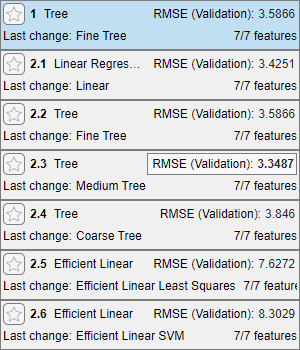• For cross-validation, the score is the RMSE on all observations not set aside for testing, counting each observation when it was in a holdout (validation) fold.

• For holdout validation, the score is the RMSE on the held-out observations.

• For resubstitution validation, the score is the resubstitution RMSE on all the training data.

The best overall score might not be the best model for your goal. Sometimes a model with a slightly lower overall score is the better model for your goal. You want to avoid overfitting, and you might want to exclude some predictors where data collection is expensive or difficult.

### View Model Metrics in Summary Tab and Models Pane

You can view model metrics in the model Summary tab and the Models pane, and use these metrics to assess and compare models. Alternatively, you can use the Results Table tab to compare models. For more information, see Compare Model Information and Results in Table View.

The Training Results metrics are calculated on the validation set. The Test Results metrics, if displayed, are calculated on an imported test set. For more information, see Evaluate Test Set Model Performance.Model Metrics

StatisticDescriptionTip
RMSERoot mean squared error. The RMSE is always positive and its units match the units of your response.Look for smaller values of the RMSE.
R-SquaredCoefficient of determination. R-squared is always smaller than 1 and usually larger than 0. It compares the trained model with the model where the response is constant and equals the mean of the training response. If your model is worse than this constant model, then R-Squared is negative.Look for an R-Squared close to 1.
MSEMean squared error. The MSE is the square of the RMSE.Look for smaller values of the MSE.
MAEMean absolute error. The MAE is always positive and similar to the RMSE, but less sensitive to outliers.Look for smaller values of the MAE.
Prediction speedEstimated prediction speed for new data, based on the prediction times for the validation data setsBackground processes inside and outside the app can affect this estimate, so train models under similar conditions for better comparisons.
Training timeTime spent training the modelBackground processes inside and outside the app can affect this estimate, so train models under similar conditions for better comparisons.
Model size (Compact)Size of the model if exported as a compact model (that is, without training data)Look for model size values that fit the memory requirements of target hardware applications.

You can sort models in the Models pane based on different model metrics. To select a metric for sorting, use the Sort by list at the top of the Models pane. Not all metrics are available for model sorting in the Models pane. You can sort models by other metrics in the Results Table (see Compare Model Information and Results in Table View).

You can also delete unwanted models listed in the Models pane. Select the model you want to delete and click the button in the upper right of the pane, click in the Models section of the Regression Learner tab, or right-click the model and select Delete. You cannot delete the last remaining model in the Models pane.

### Compare Model Information and Results in Table View

Rather than using the Summary tab or the Models pane to compare model metrics, you can use a table of results. On the Regression Learner tab, in the Models section, click . In the Results Table tab, you can sort models by their training and test results, as well as by their options (such as model type, selected features, PCA, and so on). For example, to sort models by root mean squared error, click the sorting arrows in the RMSE (Validation) column header. An up arrow indicates that models are sorted from lowest RMSE to highest RMSE.

To view more table column options, click the "Select columns to display" button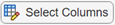at the top right of the table. In the Select Columns to Display dialog box, check the boxes for the columns you want to display in the results table. Newly selected columns are appended to the table on the right.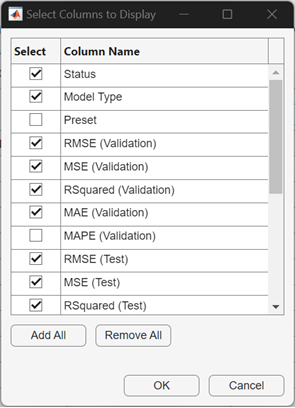Within the results table, you can manually drag and drop the table columns so that they appear in your preferred order.

You can mark some models as favorites by using the Favorite column. The app keeps the selection of favorite models consistent between the results table and the Models pane. Unlike other columns, the Favorite and Model Number columns cannot be removed from the table.

To remove a row from the table, right-click any entry within the row and click Hide row (or Hide selected row(s) if the row is highlighted). To remove consecutive rows, click any entry within the first row you want to remove, press Shift, and click any entry within the last row you want to remove. Then, right-click one of the highlighted entries and click Hide selected row(s). To restore all removed rows, right-click any entry in the table and click Show all rows. The restored rows are appended to the bottom of the table.

To export the information in the table, use one of the export buttons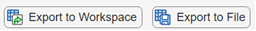at the top right of the table. Choose between exporting the table to the workspace or to a file. The exported table includes only the displayed rows and columns.

### Explore Data and Results in Response Plot

View the regression model results by using the response plot, which displays the predicted response versus the record number. After you train a regression model, the app automatically opens the response plot for that model. If you train an "All" model, the app opens the response plot for the first model only. To view the response plot for another model, select the model in the Models pane. On the Regression Learner tab, in the Plot and Interpret section, click the arrow to open the gallery, and then click in the Validation Results group. If you are using holdout or cross-validation, then the predicted response values are the predictions on the held-out (validation) observations. In other words, the software obtains each prediction by using a model that was trained without the corresponding observation.

To investigate your results, use the controls on the right. You can:

• Plot predicted and/or true responses. Use the check boxes under Plot to make your selection.

• Show prediction errors, drawn as vertical lines between the predicted and true responses, by selecting the Errors check box.

• Choose the variable to plot on the x-axis under X-axis. You can choose the record number or one of your predictor variables.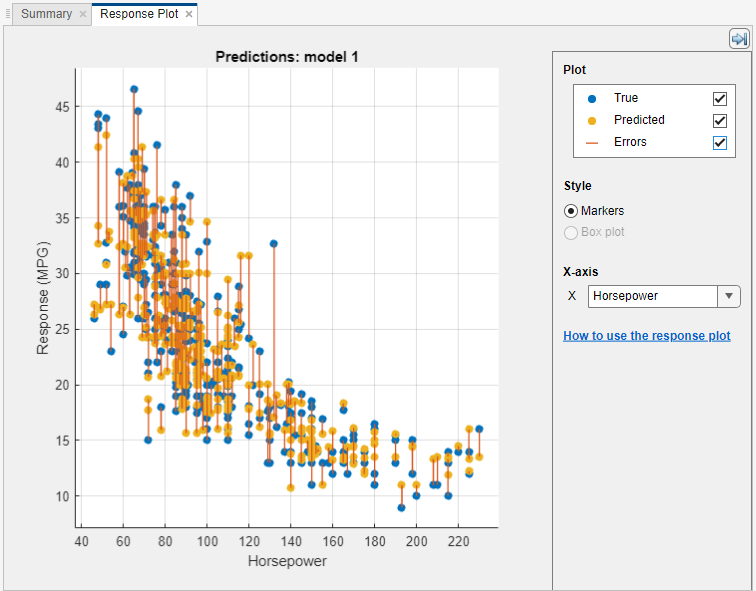• Plot the response as markers, or as a box plot under Style. You can select Box plot only when the variable on the x-axis has few unique values.

A box plot displays the typical values of the response and any possible outliers. The central mark indicates the median, and the bottom and top edges of the box are the 25th and 75th percentiles, respectively. Vertical lines, called whiskers, extend from the boxes to the most extreme data points that are not considered outliers. The outliers are plotted individually using the `"o"` symbol. For more information about box plots, see `boxchart`.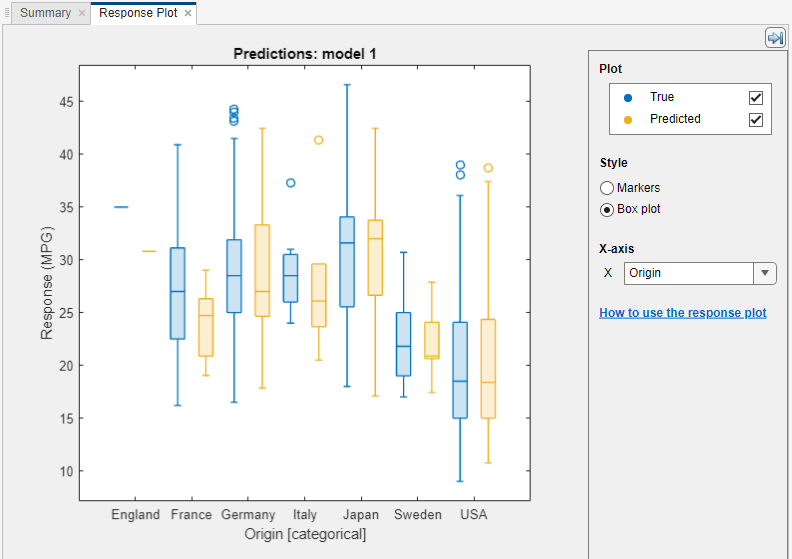To export the response plots you create in the app to figures, see Export Plots in Regression Learner App.

### Plot Predicted vs. Actual Response

Use the Predicted vs. Actual plot to check model performance. Use this plot to understand how well the regression model makes predictions for different response values. To view the Predicted vs. Actual plot after training a model, click the arrow in the Plot and Interpret section to open the gallery, and then click Predicted vs. Actual (Validation) in the Validation Results group.

When you open the plot, the predicted response of your model is plotted against the actual, true response. A perfect regression model has a predicted response equal to the true response, so all the points lie on a diagonal line. The vertical distance from the line to any point is the error of the prediction for that point. A good model has small errors, which means the predictions are scattered near the line.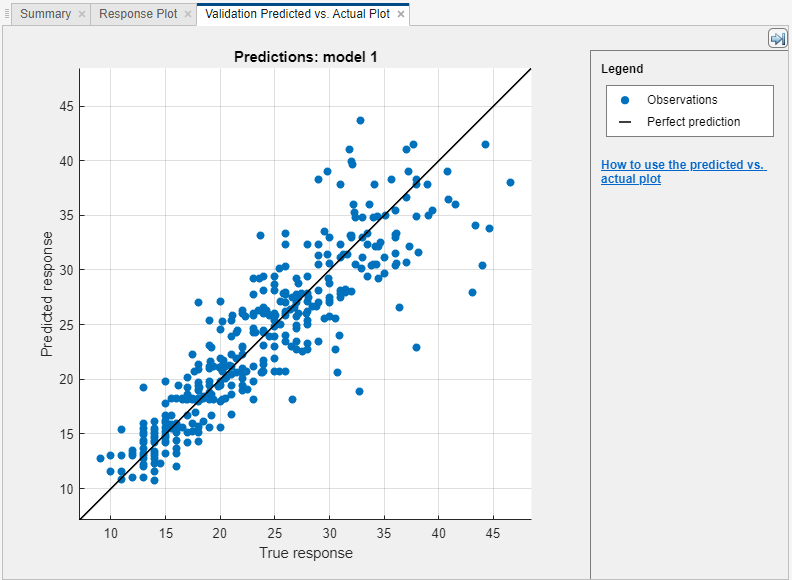Usually a good model has points scattered roughly symmetrically around the diagonal line. If you can see any clear patterns in the plot, it is likely that you can improve your model. Try training a different model type or making your current model type more flexible by duplicating the model and using the Model Hyperparameters options in the model Summary tab. If you are unable to improve your model, it is possible that you need more data, or that you are missing an important predictor.

To export the Predicted vs. Actual plots you create in the app to figures, see Export Plots in Regression Learner App.

### Evaluate Model Using Residuals Plot

Use the residuals plot to check model performance. To view the residuals plot after training a model, click the arrow in the Plot and Interpret section to open the gallery, and then click Residuals (Validation) in the Validation Results group. The residuals plot displays the difference between the predicted and true responses. Choose the variable to plot on the x-axis under X-axis. Choose the true response, predicted response, record number, or one of the predictors.Usually a good model has residuals scattered roughly symmetrically around 0. If you can see any clear patterns in the residuals, it is likely that you can improve your model. Look for these patterns:

• Residuals are not symmetrically distributed around 0.

• Residuals change significantly in size from left to right in the plot.

• Outliers occur, that is, residuals that are much larger than the rest of the residuals.

• A clear, nonlinear pattern appears in the residuals.

Try training a different model type, or making your current model type more flexible by duplicating the model and using the Model Hyperparameters options in the model Summary tab. If you are unable to improve your model, it is possible that you need more data, or that you are missing an important predictor.

To export the residuals plots you create in the app to figures, see Export Plots in Regression Learner App.

### Interpret Model Using Partial Dependence Plots

Partial dependence plots (PDPs) allow you to visualize the marginal effect of each predictor on the predicted response of a trained regression model. After you train a model in Regression Learner, you can view a partial dependence plot for the model. On the Regression Learner tab, in the Plot and Interpret section, click the arrow to open the gallery. In the Interpretation Results section, click . When computing partial dependence values, the app uses the final model, trained on the full data set (including training and validation data, but excluding test data).

To investigate your results, use the controls on the right.

• Under Data, choose whether to plot results using Training set data or Test set data. The training set refers to the data used to train the final model and includes all the observations that are not reserved for testing.

• Under Feature, choose the feature to plot using the X list. The plotted line corresponds to the average predicted response across the predictor values. The x-axis tick marks in the plot correspond to the unique predictor values in the selected data set.

If you use PCA to train a model, you can select principal components from the X list.

• Zoom in and out, or pan across the plot. To enable zooming or panning, place the mouse over the PDP and click the corresponding button on the toolbar that appears above the top right of the plot.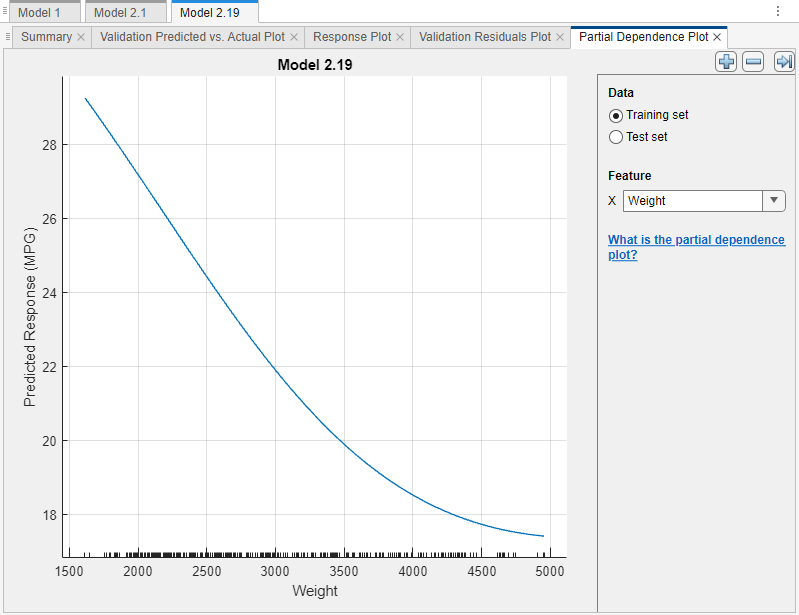For an example, see Interpret Regression Models Trained in Regression Learner App. For more information on partial dependence plots, see `plotPartialDependence`.

To export PDPs you create in the app to figures, see Export Plots in Regression Learner App.

### Compare Model Plots by Changing Layout

Visualize the results of models trained in Regression Learner by using the plot options in the Plot and Interpret section of the Regression Learner tab. You can rearrange the layout of the plots to compare results across multiple models: use the options in the Layout button, drag and drop plots, or select the options provided by the Document Actions arrow located to the right of the model plot tabs.

For example, after training two models in Regression Learner, display a plot for each model and change the plot layout to compare the plots by using one of these procedures:

• In the Plot and Interpret section, click and select Compare models.

• Click the second model tab name, and then drag and drop the second model tab to the right.

• Click the Document Actions arrow located to the far right of the model plot tabs. Select the `Tile All` option and specify a 1-by-2 layout.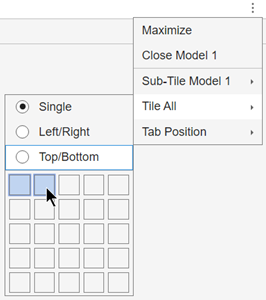Note that you can click the Hide plot options button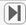at the top right of the plots to make more room for the plots.

### Evaluate Test Set Model Performance

After training a model in Regression Learner, you can evaluate the model performance on a test set in the app. This process allows you to check whether the validation metrics provide good estimates for the model performance on new data.

1. Import a test data set into Regression Learner. Alternatively, reserve some data for testing when importing data into the app (see (Optional) Reserve Data for Testing).

• If the test data set is in the MATLAB® workspace, then in the Test section on the Regression Learner tab, click and select From Workspace.

• If the test data set is in a file, then in the Test section, click and select From File. Select a file type in the list, such as a spreadsheet, text file, or comma-separated values (`.csv`) file, or select All Files to browse for other file types such as `.dat`.

In the Import Test Data dialog box, select the test data set from the Test Data Set Variable list. The test set must have the same variables as the predictors imported for training and validation.

2. Compute the test set metrics.

• To compute test metrics for a single model, select the trained model in the Models pane. On the Regression Learner tab, in the Test section, click and select Test Selected.

• To compute test metrics for all trained models, click and select Test All in the Test section.

The app computes the test set performance of each model trained on the full data set, including training and validation data (but excluding test data).

3. Compare the validation metrics with the test metrics.

In the model Summary tab, the app displays the validation metrics and test metrics in the Training Results section and Test Results section, respectively. You can check if the validation metrics give good estimates for the test metrics.

You can also visualize the test results using plots.

• Display a predicted vs. actual plot. In the Plot and Interpret section on the Regression Learner tab, click the arrow to open the gallery, and then click in the Test Results group.

• Display a residuals plot. In the Plot and Interpret section, click the arrow to open the gallery, and then click in the Test Results group.

For an example, see Check Model Performance Using Test Set in Regression Learner App. For an example that uses test set metrics in a hyperparameter optimization workflow, see Train Regression Model Using Hyperparameter Optimization in Regression Learner App.